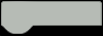# Arduino weighing machine (scale) with analog showing

This time I will show you how to make a weighing machine where the result is displayed on the Galvanometer. Instead of a Galvanometer, we can use a 5V Voltmeter, but in that case the 10 k trimmer potentiometer should be removed.

The electronic weighing scale uses a load cell which is a transducer that is used to create an electrical signal whose magnitude is directly proportional to the force being measured. This signal need to be amplified and processed, and that function is performed by a small breakout board with HX711 IC which is a 24-bit high precision A/D converter.
Usually calibrating and the final result is displayed on an LCD display or on a serial monitor, but in this case I modified the standard code so that the calibration process is displayed via LEDs and the weight is displayed on an analog instrument. In this way, in addition to the unusual Retro look it also greatly simplifies the making of the device that contains only a few components:

HARDWARE LIST
1 Arduino Nano
1 Breakout board HX711
1 Galvanometer, or 5V Voltmeter
1 Trimmer Potentiometer, 10 kohm
2 Through Hole Resistor, 470 ohm
4 LED (generic)
1 Slide SwitchAlthough the cell is intended for weighing up to 5 kg, I mapped the maximum weight to 1 kg in the code for higher resolution when measuring small weights.After switching on, the galvanometer is tested first and the arrow moves to the end position and returns. If there is a deviation from the marked scale, calibration is performed with the help of the trimmer potentiometer. Now, the blue LED lights up, indicating a process of self-calibrating the scale. During this procedure, zeroing is performed, neglecting the weight of the plate on which the measurning weight is placed. After that, the yellow Led lights up and it is a sign that you need to put a known weight that is previously defined in the code, and in our case it is 100 grams. When the calibration is complete, the white LED lights up, which means that the scale is ready for measurement.
I will also present you another case, when we need to measure the weight of an object that is in a container, without measuring the weight of the container. During the video you can see the prototype, where besides the LEDs, there is also an LCD Display which shows the current state of the scales and weight. During the construction, the LCD Display was left out due to the simplification of the project.
Finally, the device is mounted in a suitable housing made of PVC board with a thickness of 3 millimeters.CODE
``````#define DT A0
#define SCK A1

long sample=0;
float val=0;
long count=0;
int WEIGHT=0;

{
unsigned long Count;
unsigned char i;
pinMode(DT, OUTPUT);
digitalWrite(DT,HIGH);
digitalWrite(SCK,LOW);
Count=0;
pinMode(DT, INPUT);
for (i=0;i<24;i++)
{
digitalWrite(SCK,HIGH);
Count=Count<<1;
digitalWrite(SCK,LOW);
Count++;
}
digitalWrite(SCK,HIGH);
Count=Count^0x800000;
digitalWrite(SCK,LOW);
return(Count);
}

void setup()
{
pinMode(6, OUTPUT);
pinMode(7, OUTPUT);
pinMode(8, OUTPUT);
pinMode(10, OUTPUT);
pinMode(SCK, OUTPUT);

delay(500);

test();
calibrate();
}

void loop()
{
int w=(((count-sample)/val)-2*((count-sample)/val));

WEIGHT = map(w,0,1000,0,255);
analogWrite (6,WEIGHT);
}

void calibrate()
{
digitalWrite(7, HIGH);

for(int i=0;i<100;i++)

{
sample+=count;
}
sample/=100;

digitalWrite(7, LOW);
digitalWrite(8, HIGH);
count=0;
while(count<1000)
{
count=sample-count;
}

delay(2000);
for(int i=0;i<100;i++)
{
val+=sample-count;
}
val=val/100.0;
val=val/100.0; // put here your calibrating weight

digitalWrite(8, LOW);
digitalWrite(10, HIGH);
}
void test() {
for (int i=1;i<=255;i++)
{
analogWrite (6, i);
delay (15);
}
for (int i=255;i>=1;i--)
{
analogWrite (6, i);
delay (15);
}
}``````

Visit my Youtube Channel for more Projects , DIY and How To Make videos: